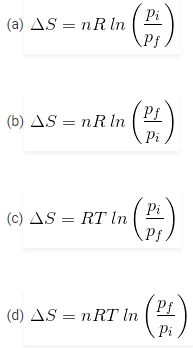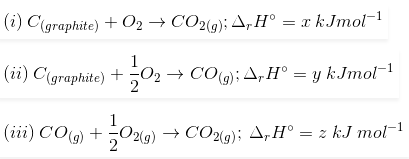# MCQs on Thermodynamics for NEET

NEET  Chemistry is the scoring paper in the medical entrance examination. Here, you will discover the NEET Chemistry MCQ Questions for all Concepts as per the latest syllabus. Practice more on a regular basis with these NEET Chemistry objective questions on air pollution and improve your subject knowledge & problem-solving skills along with time management. NEET Chemistry Thermodynamics Multiple Choice Questions make you feel confident in answering the question in the exam & increases your scores to high.

## MCQs on Thermodynamics

1. What will be the value of ΔH, if the forward and reverse reactions have the same energy of activation?
(a) ΔH = ΔG = ΔS = 0
(b) ΔS = 0
(c) ΔG = 0
(d) ΔH = 0

2. What will be the entropy change (ΔS), when an ideal gas undergoes a change in the pressure from pi to pf isothermally?3. Find out the relationship between x, y and z considering below three reactions(a) y = 2z – x
(b) x = y – z
(c) x = y + z
(d) z = x + y

4. What is the molar entropy change for melting of ice at 0℃, if ΔHf = 1.435 kcal/mol?
(a) 0.526 cal/(mol K)
(b) 5.26 cal/(mol K)
(c) 10.52 cal/(mol K)
(d) 21.04 cal/(mol K)

5. What is the function of a catalyst in a chemical reaction?
(a) decrease rate constant of reaction
(b) increases activation energy of reaction
(c) reduces enthalpy of reaction
(d) does not affect the equilibrium constant of reaction

6. What will be the work done by 3 moles of an ideal gas when it expands spontaneously in a vacuum?
(a) zero
(b) infinite
(c) 3 joules
(d) 9 joules

7. Find the temperature at which the below reaction will be in equilibrium if the enthalpy and entropy change for the reaction is 30 kJ mol-1 and 105 J K-1 mol-1 respectively

Br2(l) + Cl2(g) → 2BrCl(g)
(a) 273 K
(b) 300 K
(c) 450 K
(d) 285.7 K

8. Which is true for the entropy of a spontaneous reaction?
(a) ΔS(system) – ΔS(surroundings) > 0
(b) ΔS(system) + ΔS(surroundings) > 0
(c) ΔS(surroundings) > 0 only
(d) ΔS(system) > 0 only

9. An ideal gas is expanded isothermally at 300 K from 1 litre to 10 litres. Find the ΔE for this process (R = 2 cal mol-1 K-1)
(a) 9 L atm
(b) 1381.1 cal
(c) zero
(d) 163.7 cal